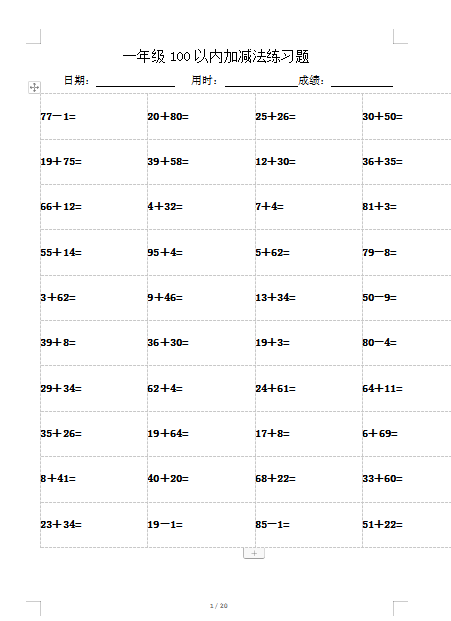77－1= 20＋80= 25＋26= 30＋50= 19＋75= 39＋58= 12＋30= 36＋35=